# CAT 2019 Question Paper | Quants Slot 1

###### CAT Previous Year Paper | CAT Quants Questions | Question 26

Exponents and Logarithms form an important part of the CAT syllabus. Logarithms require a  lot of practice as there is no intuitive way around them. The benefit of preparation is that there could be easy questions that can be attempted because of the preparation.

Question 26 : Let x and y be positive real numbers such that log5(x + y) + log5(x - y) = 3, and log2y - log2x = 1 - log23. Then xy equals

1. 25
2. 150
3. 250
4. 100

## Best CAT Coaching in Chennai

#### CAT Coaching in Chennai - CAT 2022Limited Seats Available - Register Now!

Given that, log5(x + y) + log5(x - y) = 3
We know log A + log B = log (A x B)
log5(x + y) + log5(x - y) = log5(x2 - y2)
log5(x2 - y2) = 3
x2 - y2 =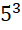x2 - y2 = 125
Similarly, log2y - log2x = 1 - log23
log2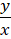= log22 - log23
log2= log2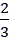3y = 2x
(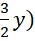2 - y2 = 1252 - y2 = 125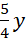2 = 125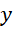2 = 100
y = 10
x = 15
So, xy = 15 x 10 = 150

The question is "Let x and y be positive real numbers such that log5(x + y) + log5(x - y) = 3, and log2y - log2x = 1 - log23. Then xy equals"

##### Hence, the answer is 150

Choice B is the correct answer.

###### CAT Coaching in ChennaiCAT 2023

Classroom Batches Starting Now! @Gopalapuram

###### Best CAT Coaching in Chennai Introductory offer of 5000/-

Attend a Demo Class

##### Where is 2IIM located?

2IIM Online CAT Coaching
A Fermat Education Initiative,
58/16, Indira Gandhi Street,
Kaveri Rangan Nagar, Saligramam, Chennai 600 093

##### How to reach 2IIM?

Mobile: (91) 99626 48484 / 94459 38484
WhatsApp: WhatsApp Now
Email: info@2iim.com